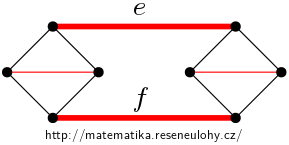## Two edges in matching

Construct a cubic graph $$G$$ without bridges different from $$K_4$$, which contains two edges of $$e$$ and $$f$$ such that G has no matching containing $$e$$ and not containing $$f$$.

In other words, every matching contains both edges or none.

• #### Hint

Consider how pairing would be affected if the graph contains a triangle.

• #### Solution

The complete graph $$K_4$$ has a unique partition into perfect matchings. Take two copes of $$K_4$$, in each choose an edge, delete these two and then add edges between vertices of degree two to make this graph connected.

The newly added edges can be extended to a unique matching, see the picture.